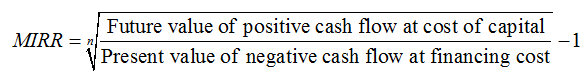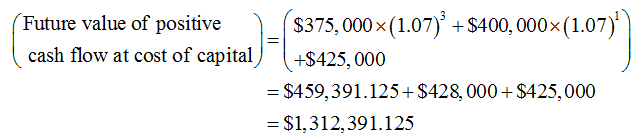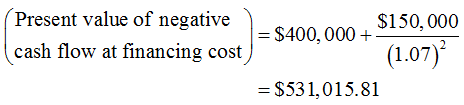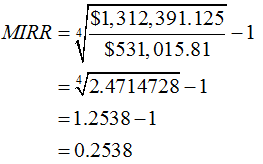# The IRR evaluation method assumes that cash flows from the project are reinvested at the same rate equal to the IRR. However, in reality the reinvested cash flows may not necessarily generate a return equal to the IRR. Thus, the modified IRR approach makes a more reasonable assumption other than the project’s IRR.Consider the following situation:Grey Fox Aviation Company is analyzing a project that requires an initial investment of \$400,000. The project’s expected cash flows are:YearCash FlowYear 1\$375,000Year 2–150,000Year 3400,000Year 4425,000 Grey Fox Aviation Company’s WACC is 7%, and the project has the same risk as the firm’s average project. Calculate this project’s modified internal rate of return (MIRR):25.38% 24.11% 29.19% 26.65% Points:    Close ExplanationExplanation: If Grey Fox Aviation Company’s managers select projects based on the MIRR criterion, they should selector 1     rejectacceptthis independent project.Points:    Close ExplanationExplanation: Which of the following statements best describes the difference between the IRR method and the MIRR method?The IRR method uses the present value of the initial investment to calculate the IRR. The MIRR method uses the terminal value of the initial investment to calculate the MIRR. The IRR method uses only cash inflows to calculate the IRR. The MIRR method uses both cash inflows and cash outflows to calculate the MIRR. The IRR method assumes that cash flows are reinvested at a rate of return equal to the IRR. The MIRR method assumes that cash flows are reinvested at a rate of return equal to the cost of capital.

Question
14 views
The IRR evaluation method assumes that cash flows from the project are reinvested at the same rate equal to the IRR. However, in reality the reinvested cash flows may not necessarily generate a return equal to the IRR. Thus, the modified IRR approach makes a more reasonable assumption other than the project’s IRR.
Consider the following situation:
Grey Fox Aviation Company is analyzing a project that requires an initial investment of \$400,000. The project’s expected cash flows are:
Year
Cash Flow
Year 1 \$375,000
Year 2 –150,000
Year 3 400,000
Year 4 425,000

Grey Fox Aviation Company’s WACC is 7%, and the project has the same risk as the firm’s average project. Calculate this project’s modified internal rate of return (MIRR):
25.38%

24.11%

29.19%

26.65%

Points:

Close Explanation
Explanation:

If Grey Fox Aviation Company’s managers select projects based on the MIRR criterion, they should selector 1

• reject
• accept
this independent project.
Points:

Close Explanation
Explanation:

Which of the following statements best describes the difference between the IRR method and the MIRR method?
The IRR method uses the present value of the initial investment to calculate the IRR. The MIRR method uses the terminal value of the initial investment to calculate the MIRR.

The IRR method uses only cash inflows to calculate the IRR. The MIRR method uses both cash inflows and cash outflows to calculate the MIRR.

The IRR method assumes that cash flows are reinvested at a rate of return equal to the IRR. The MIRR method assumes that cash flows are reinvested at a rate of return equal to the cost of capital.
check_circle

Step 1

The below expression can be used to calculated MIRR:Step 2

Future value of positive cash flow is calculated below:Present value of cash outflow is calculated below:Step 3

MIRR is calculated below:MIRR is equal to 25.38%.

...

### Want to see the full answer?

See Solution

#### Want to see this answer and more?

Solutions are written by subject experts who are available 24/7. Questions are typically answered within 1 hour.*

See Solution
*Response times may vary by subject and question.
Tagged in